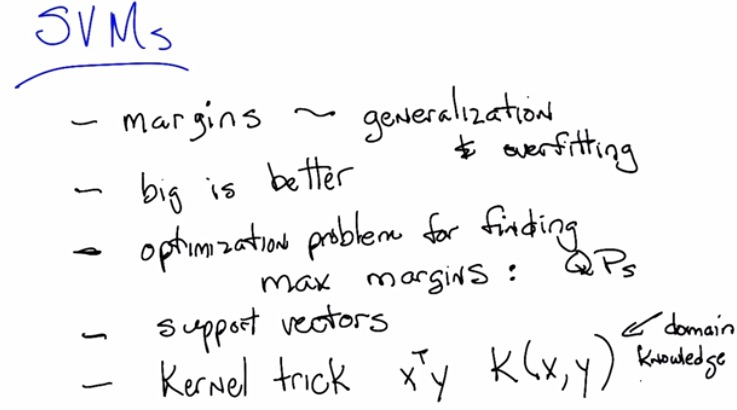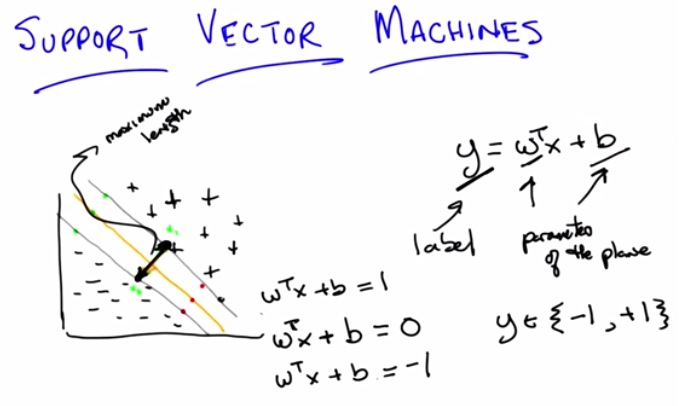• This is the SVM
• as SVM, it prefer more generalize line separator(the one in the middle), over the other, because the other tend to overfit.
• This is way SVM is less overfit over most of the algorithm
• It find a line in such a way, that the line doesn't tend to the data so much, while still maintaining its consistency.
• So we know that general function to draw line is y=mx+b
• the one above is plane function, that takes a weight parameters of x and b as the bias unit, the linear, constant value that acts to move the plane separator in and out.
• Suppose we have the binary classifier y, then we want to know the furthest distance from the data, while keeping its consistency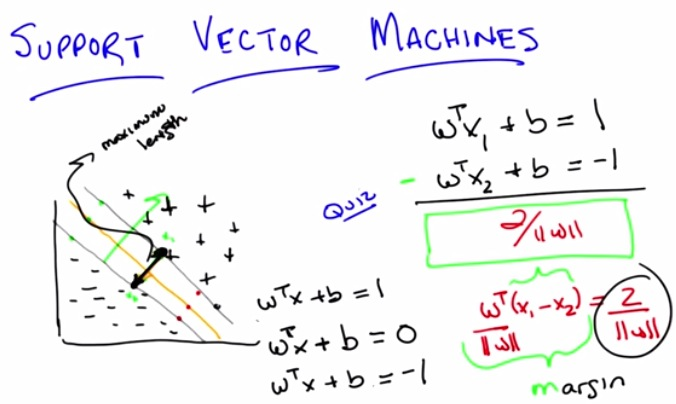• The smaller value of w, the greater the distance between x1 and x2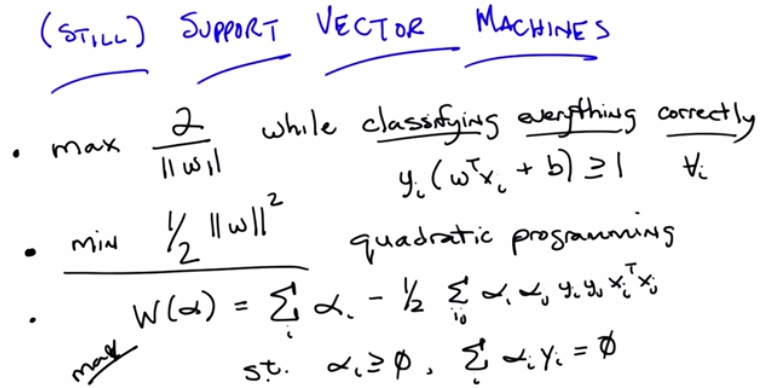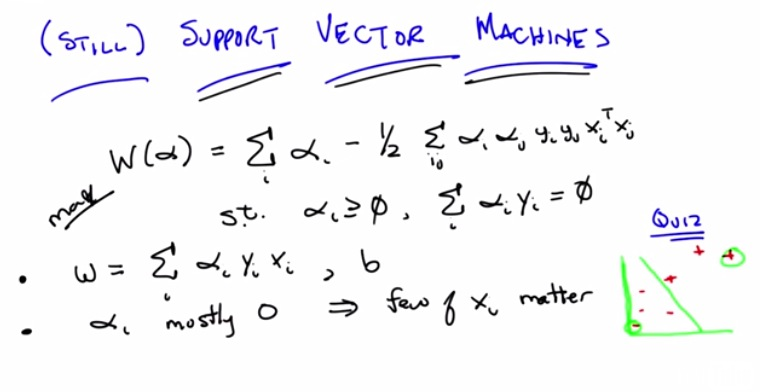• So we're doing the omega, we fetch the maximum, by using quadratic programming.
• But what matters is, because alpha tend to be zero, mostly 0, then just some of the vector, support vector , matters to the machine
• if you see at the formula, we multiply xx and yy. that is , we're using the similarity between them and count how similar, are them
• The graph on the bottom right shows that those data points are not matters for the line separator, and the equation will produce the alpha equals zero. Here we have seen some nearest neighbors, but in svm, we're using the locally weighted(just taking those within the area of separator)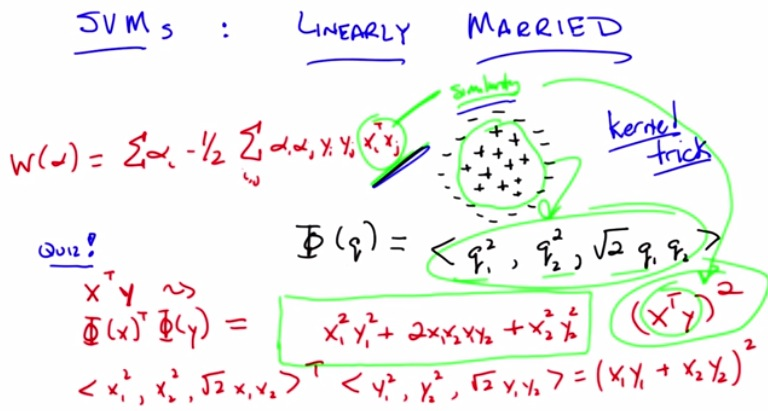• Here's what we do, we're going to fill in x and y in general phi function.
• this will return the fibonacci tree of degree 2, and simplify by XtY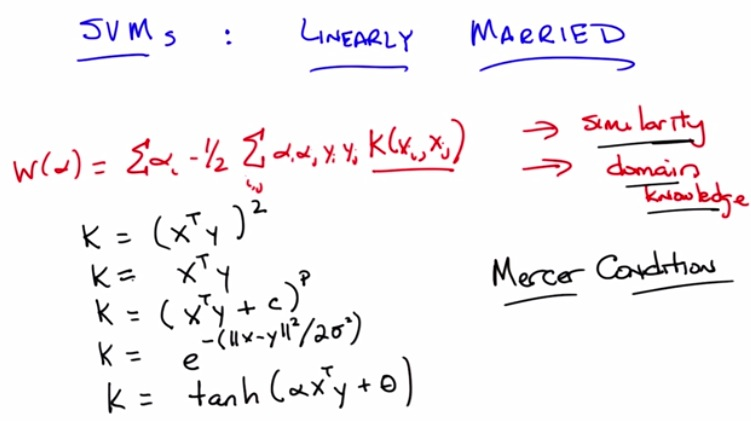• Kernel can be some arbitrary function that satisfied Mercer Condition